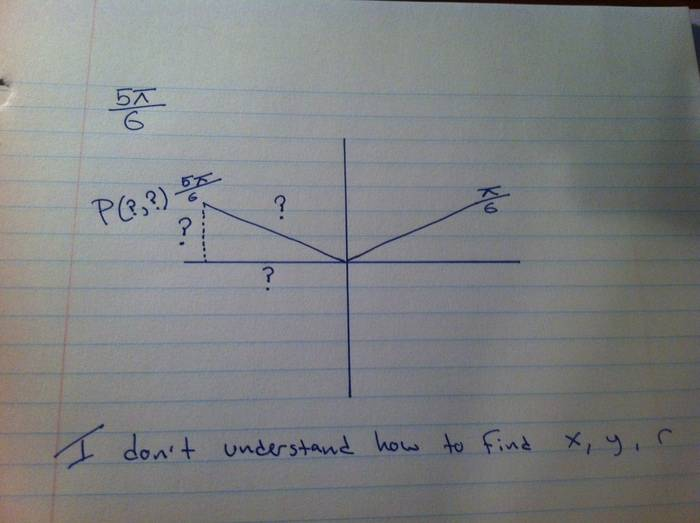# So lost on exact trig ratio questions! Please help

nukeman

## Homework Statement

I am reviewing some trig, and I forgot how to do this. Please let me figure this one simple thing out.

Here is the questions.

Find the exact trig ratios of 5∏/6

Ok, now look at my diagram below to see where I am having trouble with!What simple process am I missing?

## Answers and Replies

Homework Helper
The hypotenuse is 1, isn't it? Or at least you can assume it is, everything else being similar. The triangle you've drawn is one half of an equilateral triangle. All sides length 1, all angles pi/3. Think about it.

Last edited:
Homework Helper
The hypotenuse is 1, isn't it? Or at least you can assume it is, everything else being similar. The triangle you've drawn is one half of an equilateral triangle. All sides length 1, all angles pi/3. Think about it.

That's a refreshing way to look at the problem, but I don't think it's going to help nukeman solve other problems.

If you have a triangle with angle $\theta$ and hypotenuse length 1, then what is $\cos(\theta)$ and $\sin(\theta)$?

Homework Helper
You were probably expected to memorize these values- that's why you are lost. But it is not possible to calculate the values.

It might help to think in degrees rather than radians- many people recall geometry in terms of degrees rather than radians. $2\pi$ is a full circle as is 360 degrees: fra$\pi/6$ is the same as
$$\frac{\pi}{6}\frac{360}{2\pi}= \frac{360}{12}= 30$$.

Now look at an equilateral triangle. In any triangle, the angles add to 180 degrees ($\pi$ radians) and in an equilateral triangle all three angles are equal so each is 180/3= 60 degrees ($\pi/3$ radians). Now draw a perpendicular from one vertex to the opposite side. That also divides both the angle and the side into equal parts into equal parts, forming a right triangle with angles of $\pi/3$ and $\pi/6$. If we take the sides of the original equilateral triangle to be 2, that is the hypotenuse of a right triangle and the leg opposite the $\pi/6$ angle is 1. By the Pythagorean theorem, the the other leg has length $\sqrt{2^2- 1^2}= \sqrt{3}$. Then
$$sin(\pi/6)= \frac{\sqrt{3}}{2}$$
$$cos(\pi/6)= \frac{1}{2}$$
etc.